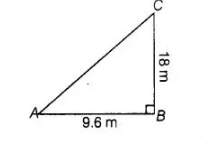# A flag pole 18 m high casts a shadow 9.6 m long.

Question:

A flag pole 18 m high casts a shadow 9.6 m long. Find the distance of the top of the pole from the far end of the shadow.

Solution:

Let BC = 18 m be the flag pole and its shadow be AB = 9.6 m. The distance of the top of the pole, C from the far end i.e., A of the shadow is AC.In right angled $\triangle A B C, \quad A C^{2}=A B^{2}+B C^{2} \quad$ [by Pythagoras theorem]

$\Rightarrow \quad A C^{2}=(9.6)^{2}+(18)^{2}$

$A C^{2}=92.16+324$

$\Rightarrow \quad A C^{2}=41616$

$\therefore \quad A C=\sqrt{41616}=20.4 \mathrm{~m}$

Hence, the required distance is 20.4 m.